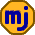## Thursday, January 30, 2020

### TD to Start Charging More Interest on Credit Cards

Recent reports that TD will start charging compound interest on all personal credit cards are only partially true.  TD was charging some compound interest on these credit cards and will start charging more.

The relevant section of the credit card agreement used to read as follows:

If interest is charged, it is calculated on the average daily balance of each Transaction from the transaction date until that amount is paid in full.  The total is the amount of interest we will charge you on each statement on the last day of your statement period.

The new agreement replaces the last sentence with the following:

We add your unpaid interest charge to your balance at the end of each statement period.  As a result, we charge interest on unpaid interest.

The difference is in the time from the end of a statement period until the due date for your payment.  During this time on certain personal credit cards, TD is now charging daily interest on the newly accumulated interest instead of giving you a few weeks interest-free to pay this interest.  Keep in mind that if you haven’t paid your balance in full, they’ve always charged daily interest on the rest of your balance during the time from the statement end to the payment date. It’s just the newly accumulated interest that is treated differently.

So, what does all this mean for the amount of interest TD charges?  On the surface, it seems like a change from simple interest to compound interest, but this isn’t right.  Under the old rules, there is compounding of interest upon interest with a delay. This may not seem right if you pay off the interest each month, but it’s still compound interest.  If it were truly simple interest, you could wait until the end of the year to pay all the interest without any additional interest being charged.

I’ll leave the details to the end of this post for the few who may be interested, but if a credit card charges a nominal interest rate of 20%, the compounded interest rate under TD’s old rules is 21.59%.  The calculation is simpler under the new rules because the full debt pays interest every day and gets compounded monthly. So, we take one-twelfth of 20% and compound it 12 times to get 21.94%.

So, we see that TD was already getting most of the compounding before they changed the rules, and now they get all of it.  We might wonder why they would bother making this change for so little benefit. Big banks are under pressure to keep their dividend payments growing, and they won’t leave any stone unturned in their search for higher profits.

The Gory Math Details

Let r be the monthly nominal interest rate: r=20%/12.  Let t be the fraction of a month delay from the end of a statement period to the payment date.  On one of my credit cards, this is 3 days short of one month: t=1-3/(365/12). If TD’s delay is different, it will make a small difference to this calculation.

Let x be the rate of increase of a credit card debt from one payment date to the next.  This is the quantity we want to calculate. So, without any intervening payments, the debt in 3 successive months is M, M(1+x), M(1+x)(1+x).

The interest in the last month is M(1+x)(1+x)-M(1+x)=Mx(1+x).  We can also calculate this last month’s interest a different way.  Before the payment date, interest accumulates on a debt of M for a total of Mrt.  After the payment date, interest accumulates on M(1+x) for a total of M(1+x)r(1-t).

Equating the two interest totals gives the quadratic equation xx+(1-r+rt)x-r=0.  We can solve this for x and then calculate the compounded annual interest rate (1+x)^(12)-1.  For the numbers in our example, this works out to 21.59%.

After TD’s rule change, the compounded interest rate is (1+r)^(12)-1=21.94%.

1.2.